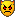### using vector2rot.mel ?#256183

By deadalvs
#256183
i use this script to get the rotation in xyz to get from the up-vector to a desired direction...
(http://www.highend2d.com/boards/lofiver ... 16269.html)

the question is how to apply the resulting float array values from the script so that the rotation actually gives a correct value !the example shows a scaled locator that should go thru the diagonal !
polyCube -w 1 -h 1 -d 1 -sx 1 -sy 1 -sz 1 -ax 0 1 0 -cuv 4 -ch 1;
setAttr "pCube1.translateX" .5;
setAttr "pCube1.translateY" .5;
setAttr "pCube1.translateZ" .5;
spaceLocator -p 0 0 0;
setAttr "locator1.scaleX" .2;
setAttr "locator1.scaleY" .2;
setAttr "locator1.scaleZ" .2;
setAttr "locator1.scaleY" 5;

vector \$up = <<0,1,0>>;
vector \$dir = <<1,1,1>>;

float \$rotationArray[] = vector2rot (\$dir, \$up);
rotate -r (\$rotationArray) 0 0;
rotate -r 0 (\$rotationArray) 0;
rotate -r 0 0 (\$rotationArray );

global proc float[] vector2rot( vector \$direction, vector \$up ) {

// normalize vectors first
\$direction = unit( \$direction );
\$up = unit( \$up );

// calculate the side vector
vector \$sideVec = cross( \$up, \$direction );
vector \$upVec = cross( \$direction, \$sideVec );

float \$cosY = sqrt( (\$sideVec.x)*(\$sideVec.x) + (\$sideVec.y)*(\$sideVec.y) );

// solve each angle
float \$rotZ = atan2( (\$sideVec.y), (\$sideVec.x) );
float \$rotY = atan2( -(\$sideVec.z), ( ((\$sideVec.x)+(\$sideVec.y)) / (cos( \$rotZ ) + sin( \$rotZ ) ) ) );
float \$rotX = atan2( (\$upVec.z), (\$direction.z) );

// convert to degrees
\$rotX = rad_to_deg( \$rotX );
\$rotY = rad_to_deg( \$rotY );
\$rotZ = rad_to_deg( \$rotZ );

// return x,y,z rotations
return { \$rotX, \$rotY, \$rotZ };

} // vector2rot

### #256185

By deadalvs
#256185
or has anyone a script that does the similar ?
###### Maya plug-in MR 5.2

For instance, I'm pretty sure that people would […]

###### FILE EXPORTATION FROM RHINO TO STUDIO

Hello Paolo, If you prefer not to buy the plugin […]

###### Update

Welcome back Ivox! It's nice to see you around. […]

###### Help with plastic/glass material

One more test to add. This time with a created HD[…]